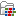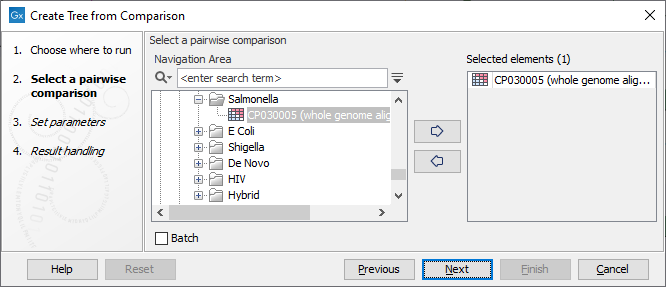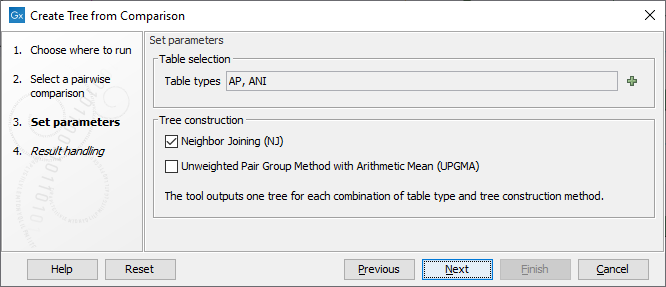# Create Tree from Comparison

The Create Tree from Comparison tool builds a tree from a Pairwise Comparison such as those generated by Create Average Nucleotide Identity Comparison tool.

To run the Create Tree from Comparison tool:

Toolbox | Whole Genome Alignment () | Create Tree from Comparison ()

Once the tool wizard has opened (figure 5.1), choose the Pairwise Comparison table you would like to use.Figure 5.14: Select a Pairwise Comparison table.

In the next dialog (figure 5.2), you can set the following parameters:Figure 5.15: Select the table types and tree construction methods you would like to use for building trees.

• Table types The possible table types are extracted from the Pairwise Comparison table input. In the case of a Pairwise Comparison table obtained from Create Average Nucleotide Identity Comparison, these are: ANI (Average Nucleotide Identity) or AP (Alignment Percentage). If left empty, as it is by default, both types will be used.
• Tree construction method Neighbor Joining, well suited for trees with varying rates of evolution, and / or UPGMA, method that assumes a constant rate of evolution. Learn more about distance based reconstruction methods here: http://resources.qiagenbioinformatics.com/manuals/clcgenomicsworkbench/current/index.php?manual=Distance_based_reconstruction_methods.html.

Note that the tool outputs a tree for each combination of table types and tree construction method. In addition, metadata from the Pairwise Comparison is automatically transferred to the tree. Sequence metadata containing taxonomy information is also added if this information was present in the inputs.

`d[i][j] = (t[i][j] + t[j][i]) / 2` if the table is a distance matrix,
`d[i][j] = (1 - t[i][j] + 1 - t[j][i]) / 2` if the table is a similarity matrix,
where `t[i][j]` is the relative value (between 0 and 1) found in the table in row i and column j.